# Thread: Related Rates and Tangents?

1. ## Related Rates and Tangents?

1) So this is our first hw with related rates and I am having some trouble with this one. It is basically a right triangle, with the horizontal, or x, equalling 100. It says that a person standing at point A (furthest left point) is watching a balloon as it rises from point C to point B (lowest right to highest right). The balloon is moving at a constant rate of 5 m/s.
a) Find the rate of change of z (hypotenuse, between B and A) at the instant when the balloon (y) is 50 feet high (y=50).
b) Find the rate of change in the area of right triangle BCA at the instant when y=50.
c) Find the rate of change in theta ( angle A) at the instant when y=50.

2) Let f(x)=12-x^2 for x> or equal to 0.
a) the line tangent to the graph of f at point (k, f(k)) intercepts the x-axis at x=4. What is the value of k?
b) An isosceles triangle whose base is the interval form (0,0) to (c,0) has its vertex on the graph of f. For what value of c does the triangle have maximum area? Justify.

If anyone can help with any of this let me know, thanks. Even if you can just guide me, I know the guidlines for related rates but it isn't making sense to me. Thanks

2. Hey, it must have been a chain rule balloon!

OK, 1).

Related rates nearly always depend on the chain rule, so you might want to try filling up this pattern...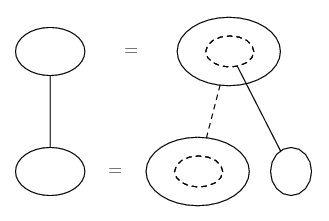... where straight continuous lines differentiate downwards (integrate up) with respect to the main variable (in this case time), and the straight dashed line similarly but with respect to the dashed balloon expression (the inner function of the composite which is subject to the chain rule).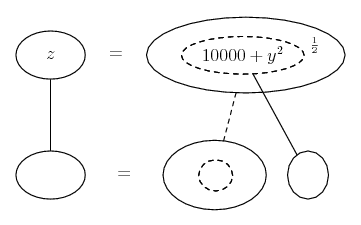So differentiate with respect to the inner function, and the inner function with respect to t, then sub in the given values of y and dy/dt...

Spoiler: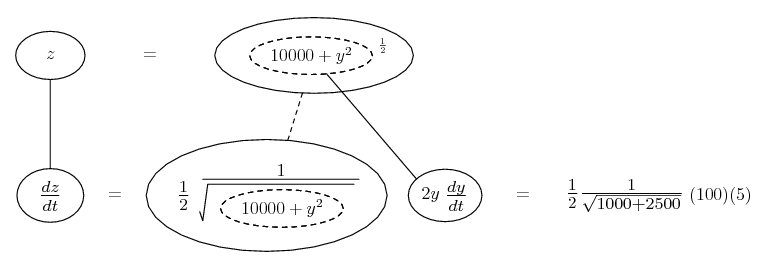Or, so as not to skirt the issue of the chain rule applying to y^2 itself, you might prefer...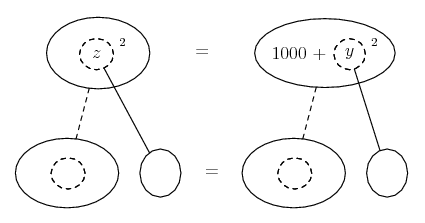Spoiler: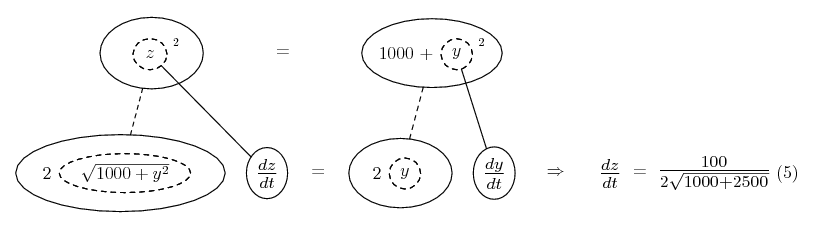OK, let's use the area formula in the same way...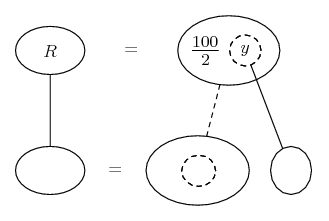Spoiler: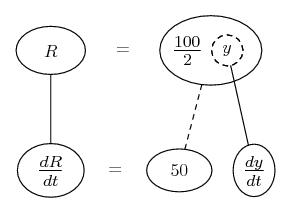For the angle A, I would do...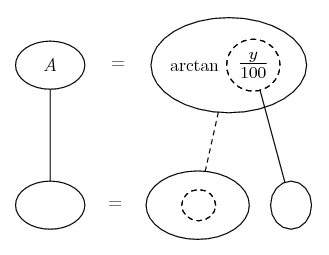Spoiler: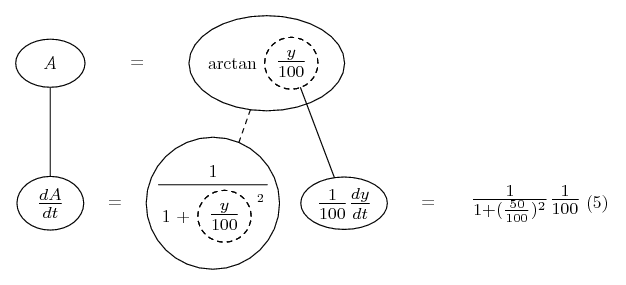Though, you may prefer to put tan A = y/100 on the top level, analogous to the alternative I gave for part a).

No 2.

One of many ways to do part a) is to meditate on our sketch (having drawn one!) and think, what do we know, and what are we trying to find...

Well, we know the slope of the tangent is -2k... and we can see that one particular example of this slope is a drop of 12 - k^2 over a horizontal progress of 4 - k. So...

$\displaystyle -2k = -\frac{12 - k^2}{4 - k}$

Solve for k.

b) Express area A as a function of c. Differentiate this function and set the derivative to zero. Solve for c.

_________________________________________

Don't integrate - balloontegrate!

Balloon Calculus: Standard Integrals, Derivatives and Methods

Balloon Calculus Drawing with LaTeX and Asymptote!

#### Search Tags

rates, related, tangents